# APPLICATION IN SQUARING

## Polynomial of Class 9

### TERMS AND THEIR COEFFICIENTS:

If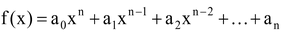is a polynomial in variable x, then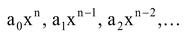and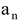are known as the terms of polynomial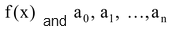and respectively are known as their coefficients, where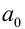is non-zero quantity and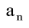is called the constant term.

In the polynomial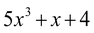the coefficient of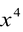is 0 whereas the constant term is – 8.

question Write the coefficients of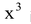in each of the following.

(i)(ii)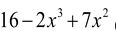(iii)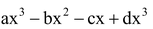Solution: (i) Coefficient of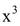is 5.

(ii) Coefficient ofis –2.

(iii) Polynomial can be written as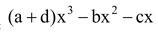coefficient of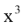is a + d.

question Write the coefficients of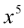in each of the following.

(i)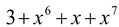(ii)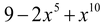Solution: (i) Coefficient of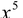is 0.

(ii) Coefficient ofis –2.

### DEGREE OF THE POLYNOMIAL:

Highest Index of x in algebraic expression is called the degree of the polynomial, here a0, a1x, a2x2 .....anxn, are called the terms of the polynomial and a0. a1, a2......, an are called various coefficients of the polynomial f(x).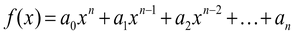In the given expression if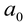is non zero then this polynomial has degree n.

Things to remember - A polynomial in x is said to be in standard form when the terms are written either in increasing order or decreasing order of the indices of x in various terms.

e.g.

1.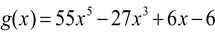highest degree of this polynomial is 5.

2.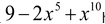highest degree of this polynomial is 10.

3.highest degree of this polynomial is 3.